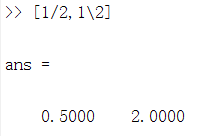• MATLAB除法运算的精度问题及其解决方案1. 问题描述：2. 解决方案1（roundn）3. 解决方案2（除变乘）4. 有更多想法或者问题，欢迎多多交流 1. 问题描述： 今天做课题的时候，发现MATALB在执行除法运算时，如果需要的...


MATLAB除法运算的精度问题及其解决方案
1. 问题描述：2. 解决方案1（roundn）3. 解决方案2（除变乘）4. 有更多想法或者问题，欢迎多多交流

1. 问题描述：
今天做课题的时候，发现MATALB在执行除法运算时，如果需要的精度太高，会出现高精度部分运算出错的情况。
比如在这里我执行一个简单的除法运算：x=4.8/0.2，看一下结果：
命令行的结果看起来还不错，24.0000，就是24嘛！打开变量查看器查看，就会发现，嗯？结果差了0.000000000000004。有时候这一点点精度缺失确实不值得注意，但是偶尔它也会产生很多麻烦，因此还是解决一下比较好。
2. 解决方案1（roundn）
roundn(X,N) 函数的作用是将X圆整，参数N定义圆整的精度，N为正代表圆整到小数点前N位，N为负代表圆整到小数点后N位。
这并不是一个很合理的解决方案，因为圆整操作会导致数值精度的丢失，但是至少可用。
我们使用： x=roundn(4.8/0.2,-10) ,执行一下，看效果：
命令行的结果其实已经表明我们的计算结果没问题了，确实是24。再打开变量查看器，发现结果确实是正确的。这个方案损失了精度，所以对于精度要求高的场合，并不适用。
3. 解决方案2（除变乘）
为了不损失精度，我们可以将除法转换为乘法，经测试也可以解决。
这里我们使用式子：x=4.8*（1/0.2），看一下命令行的结果：4. 有更多想法或者问题，欢迎多多交流
展开全文精度
• 有许多方法可以评估Z逆变换。 其中之一是通过长除法进行的逆 Z 变换。 在这里，我提交了一个函数来轻松做到这一点。 一个多项式可以任意次除以任意多项式任意次。 代码最初是在2000年编写和上传的。
• 详细介绍了MATLAB中的矩阵乘除法用法，使MATLAB编程不在迷惑、
• MATLAB计算除法的GUI程序，做MATLAB GUI练习时编的。
• 用图像除法完成减背景操作（运用公式：f=g ÷ b х m ）。
• ## Matlab除法结果取整

万次阅读 2016-01-03 17:28:01
1)fix(x) : 截尾取整. >>fix(37/10) ans =  3 (2)floor(x):不超过x 的最大整数.(高斯取整) >>floor(5.6) ans =  5 (3)ceil(x) : 大于x 的最小整数 >> ceil(4/6) ...>>ro
1)fix(x) : 截尾取整.

>>fix(37/10)

ans =

3

(2)floor(x):不超过x 的最大整数.(高斯取整)
展开全文• 本资源用MATLAB实现求已知阶数多项式是否为不可约多项式。其中可以设置阶数最大值，即可求出该阶数以内的所有不可约多项式。本程序中涉及到二进制的加法，除法运算，以及二进制与十进制，矩阵，字符串之间的转换。
• 在学习过程中发现matlab中的两种除法比较容易让人混淆，也很难记住，即左除和右除。 在matlab中运行下列语句，可以发现，两种除法的结果是不一样的。1/2的结果是0.5，但是1\2的结果却是相反的2。 其实可以发现了...
目前正在学习matlab。在学习过程中发现matlab中的两种除法比较容易让人混淆，也很难记住，即左除和右除。  在matlab中运行下列语句，可以发现，两种除法的结果是不一样的。1/2的结果是0.5，但是1\2的结果却是相反的2。其实可以发现了，1\2左右对称一下，其实就是2/1，\其实就是可以认为普通除法的方向换了一下。
这一点也在求解线性方程组中得到了验证。  左除：


展开全文• 第二讲 MATLAB的矩阵及数组运算;内容提要;矩阵和数组是MATLAB最基本的部分矩阵和数组运算又是MATLAB最基本的功能实际上MATLAB是以矩阵为基本的运算单元向量和标量作为特殊的矩阵来处理向量看作是只有一行或一列的...
• matlab开发-用长除法计算变压器。用长除法计算逆Z变换。
• ## matlab中的乘除法

万次阅读 多人点赞 2016-12-16 17:56:04
matlab中的乘除法： 1.数字之间相乘/相除 >> a=3; >> b=4; （1）相乘（*与.*没区别）： >> a*b ans =  12 >> a.*b ans =  12 （2）相除（/与./没区别）： >> a=3; >> b=4; >> a/b ans =  0...
matlab中的乘除法：
1.数字之间相乘/相除
>> a=3; >> b=4;
（1）相乘（*与.*没区别）：
>> a*b ans =     12  >> a.*b ans =     12
（2）相除（/与./没区别）：
>> a=3; >> b=4; >> a/b ans =     0.7500 >> a./b（a除以b） ans =     0.7500
>> a.\b（b除以a） ans =     1.3333

2.数字与矩阵之间相乘/相除
>> a=3; >> A=[1,3,5;3,4,5;2,4,8];
A =      1     3     5      3     4     5      2     4     8
（1）相乘（*与.*没区别）： >> a*A ans =      3     9    15      9    12    15      6    12    24 >> a.*A ans =      3     9    15      9    12    15      6    12    24
（2）相除（/与./没区别）：
>> A/a（矩阵A中元素都除以a，输出矩阵） ans =     0.3333    1.0000    1.6667     1.0000    1.3333    1.6667     0.6667    1.3333    2.6667 >> A./a ans =     0.3333    1.0000    1.6667     1.0000    1.3333    1.6667     0.6667    1.3333    2.6667
>> A.\a（a除以矩阵A中各元素，输出矩阵） ans =     3.0000    1.0000    0.6000     1.0000    0.7500    0.6000     1.5000    0.7500    0.3750

3.矩阵之间相乘/相除
>> A=[1,3,5;3,4,5;2,4,8] A =      1     3     5      3     4     5      2     4     8 >> B=[2,5,8;3,7,2;5,3,2] B =      2     5     8      3     7     2      5     3     2
（1）相乘：
>> A*B（矩阵乘法：要求左矩阵的行数与右矩阵的列数相等，即MxN维矩阵乘以NxM维矩阵。按照矩阵相乘规则计算结果）

ans =     36    41    24     43    58    42     56    62    40
>> A.*B（矩阵点乘：要求两矩阵维数相等，即MxN维矩阵乘以MxN维矩阵。矩阵对应位置元素相乘输出） ans =      2    15    40      9    28    10     10    12    16 （2）相除：
>> A/B（相当于A乘以B的逆，如下所示） ans =     0.6395   -0.0058   -0.0523     0.5233    0.0407    0.3663     1.0233   -0.2093    0.1163
>> A*inv(B) ans =     0.6395   -0.0058   -0.0523     0.5233    0.0407    0.3663     1.0233   -0.2093    0.1163 >> A./B(矩阵点右除：要求两矩阵维数相等，即MxN维矩阵除以MxN维矩阵。矩阵对应位置元素相除输出，A矩阵对应元素除以B矩阵对应元素) ans =     0.5000    0.6000    0.6250     1.0000    0.5714    2.5000     0.4000    1.3333    4.0000
>> A\B（相当于A的逆乘以B，如下所示） ans =     1.3000   -1.7000   -7.8000    -1.6000    5.4000    9.6000     1.1000   -1.9000   -2.6000
>> inv(A)*B ans =     1.3000   -1.7000   -7.8000    -1.6000    5.4000    9.6000     1.1000   -1.9000   -2.6000 >> A.\B(矩阵点左除：要求两矩阵维数相等，即MxN维矩阵除以MxN维矩阵。矩阵对应位置元素相除输出，B矩阵对应元素除以A矩阵对应元素) ans =     2.0000    1.6667    1.6000     1.0000    1.7500    0.4000     2.5000    0.7500    0.2500 
展开全文• 在矩阵中表示逆 所以若A矩阵为非奇异方阵，则存在逆矩阵，可利用inv求逆：inv(A) 我们先从符号上区分右 / 和左 \，把 / 和 \ 看成一根木棍，木棍向右倒 / 称为右，木棍向左倒 \ 称为左。 假设A矩阵是非奇异...1024程序员节 线性代数
• 1、* 和 .* 的区别 （1）* 就是通常我们在线性代数中使用的矩阵乘法的符号...如果a或b是一个标量，那么matlab会将该标量值扩展到适当大小的数组中。 x=ldivide（b，a）是将a除以b的另一种方法，但很少使用。
• MATLAB除法运算分为右除和左除。 MATLAB的算术运算是在矩阵意义下进行的。 单个数据的算术运算只是矩阵运算的一种特例。 （1）加减运算 若两矩阵同型，则运算时两矩阵的相应元素相加减。 若两矩阵不同型，则MA
• 简单几行代码，本代码主要利用MATLAB工具实现浮现数的运算，简单明了，易于理解。
• 实现去卷积和多项式除法 poly 求具有指定根的多项式 polyder 多项式求导 polyeig 求多项式的特征值 polyfit 求多项式的拟合 polyint 解析多项式积分 polyval 按数组运算规则计算多项式值 polyvalm 按...
• 正在使用广义欧几里得除法运行计算（520，3344），运算过程如下： 3344 = 6 · 520 ＋ 224 520 = 2 · 224 ＋ 72 224 = 3 · 72 ＋ 8 72 = 9 · 8 ＋ 0 经运算， 520 和 3344 的最大公因数为 8 。 拓展...
• 二元多项式除法电路原理 例：g(x)=x4+x2+x+1g(x)=x^4 + x^2+x+1g(x)=x4+x2+x+1，a(x)=x5+x3+1a(x) = x^5+x^3+1a(x)=x5+x3+1，寄存器个数等于g(x)g(x)g(x)次数为4，a(x)a(x)a(x)除以g(x)g(x)g(x)的运算过程如下： g...多项式 信道编码
• Matlab提供了两种除法运算：左除（\）和右除（/）。 一般情况下，x=a\b是方程a*x =b的解，而x=b/a是方程x*a=b的解。 例： 如果a为非奇异矩阵，则a\b和b/a可通过a的逆矩阵与b阵得到： a\b = inv(a)*b b/a = b*inv...
• 分子在上分母在下，也可以把它当做除法来看，用分子除以分母(因0在除法不能做除数，所以分母不能为0(例，表示把单位“1”平均分0份，取10份，完全没有意义))相反除法也可以改为用...全部 分式：定义 形如，A、B是...python broadcast
• matlab中的乘除法： 1.数字之间相乘/相除 >> a=3; >> b=4; （1）相乘（*与.*没区别）： >> a*b ans =     12 >> a.*b ans =     12 ...
• Find the quotient and remainder whenx^3 - x*y^2 + 1is divided byx + y. syms x y p = x^3 - x*y^2 + 1; d = x + y; [r,q] = polynomialReduce(p,d) r = 1 q = x^2 - y*x图像处理
• 1、环境要求：Windows2000/XP/7；C；信息交换内容为文本文件；信息交换方式为共享文件 2、编码要求：生成多项式为CRC-32 3、功能要求：能在两台计算机机上运行程序，一台产生CRC码，另一台校验CRC码
•Z变换
• 包含文档和代码 分别用高斯消元和列主元消去编程求解方程组 ，并分别求出矩阵A的LU分解及列主元的LU分解（求出L，U，P），并用LU分解的方法求A的逆矩阵及A的行列式
• 目录一、问题引出与求证二、问题查阅与解决三、MATLAB如何修改计算结果保留的有效数字四、拓展——数值显示四、后记 一、问题引出与求证 今天，利用MATLAB编写乘同余法产生随机数相关代码时，遇到一个问题： （下图......

# matlab除法计算matlab 订阅